# Catch up on the latest AI articles# Comparing Prior Learning Language Models In Continuous Learning

3 main points
✔️ Apply continuous learning methods to pre-learning language models
✔️ Compare the robustness of each model and continuation learning method
✔️ Analyze pre-trained language models for each layer

Pretrained Language Model in Continual Learning: A Comparative Study

The images used in this article are from the paper, the introductory slides, or were created based on them.

## first of all

Continual learning, in which models are learned while avoiding catastrophic forgetting, has been studied extensively and many methods have been proposed. In the field of natural language processing, many pre-training models such as BERT and ALBERT have been proposed and actively studied.

This article presents a comprehensive comparative study of the performance of a combined continuous learning and prior learning model and describes a paper that reveals a variety of information about continuous learning using a prior learning model.

## introduction

First, we introduce the prerequisites for the comparison experiments, such as pre-trained language models, continuation learning settings, and continuation learning methods.

### Pre-Learned Language Models (PLM)

The use of pre-trained language models (PLMs) is an effective method for many NLP tasks such as question answering and summarization. In the paper, we use the following five pre-trained language models

• GPT-2: An autoregressive language model that predicts one token at a time from left to right, and is often used for natural language generation tasks.

For more details, please refer to the original paper or the commentary article on this website.

### Setting the Continuous Learning Problem

Continuous learning aims to learn a given set of tasks without degrading the performance of previously learned tasks. It is commonly evaluated in an incremental (incremental) classification task setting at learning time. More specifically, three settings are used: class, domain, and task incremental learning.

In any learning setting, the continuous learning algorithm is given each task once in turn, but the following differences exist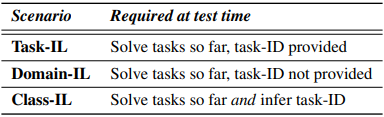• Domain-IL: At test time, no information about the task to be processed (task-ID) is given, but there is no need to predict information about the task being processed.

This setup follows previous studies.

### Continuous Learning Methodology

Continuous learning methods can be classified into three main categories (rehearsal, regularization, and dynamic architecture).

Rehearsal-based method

In rehearsal-based methods, past samples are stored in some form and reused when training for new tasks. The simplest method is to replay past samples with new data to suppress catastrophic forgetting. This is known as Experience Replay (ER) and is often an advanced baseline.

Many rehearsal-based methods have been proposed to improve the performance and efficiency of ER, such as constrained optimization using past samples instead of simple replay to prevent increased losses in past tasks.

regularization-based method

Regularization-based methods address catastrophic forgetting by preventing significant updates of parameters deemed important in previous tasks. These methods start with Elastic-Weight Consolidation (EWC ), where EWC suppresses large changes in previously learned weights using L2 regularization loss.

Regularization-based methods tend to rely on task boundaries and often fail in settings where long task sequences or task IDs are not given.

Dynamic Architecture Methodology

Dynamic architecture methods also called parameter separation methods, utilize different subsets of model parameters to fit different tasks. Some well-known methods that fall into this category include Hard Attention to the Task (HAT).

Similar to the regularization-based method, the regular dynamic architecture method requires a task ID at test time.

## experiment

### Continuous learning of PLM through benchmarking

The experiment will investigate three questions

• (1) Does the problem of catastrophic forgetting exist during continuous learning of PLM?
• (2) Which continuous learning method is the most efficient in PLM?　What are the reasons?
• (3) Which PLM is the most robust against continuous learning?　What are the reasons for this?

### experimental setup

In our experiments, we combine PLM and linear classifiers to compare the following methods.

• Vanilla: The model learned in the previous task is directly optimized for the next task. This is the setting where catastrophic forgetting is most likely to occur and corresponds to a weak lower bound.
• Joint: All tasks are learned simultaneously. It is not affected by catastrophic forgetting, so it is the upper bound of performance.
• EWC: We use EWC, which is a regularization-based method.
• HAT: Use HAT, a dynamic architecture method. (Not applicable in Class-IL configuration)
• ER: We use ER, which is a rehearsal-based method.
• DERPP: We use DERPP, a hybrid rehearsal-regularization-based method.

The evaluation indicators are as follows

• Forward transfer:$FWT=\frac{1}{T-1}\sum^{T-1}_{i=2}A_{T,i}-\tilde{b_i}$
• Backward transfer:$BWT=\frac{1}{T-1}\sum^{T-1}_{i=1}A_{T,i}-A_{i,i}$
• Average accruacy:$Avg.ACC=\frac{1}{T}\sum^T_{i=1}A_{T,i}$

where $A_{t,i}$ is the test accuracy of the $i$th task after training the model on the $t$th task and $\tilde{b_i}$ is the test accuracy of the $i$th task on random initialization.

The dataset used in the experiment is as follows

• CLINC150
• MAVEN.
• WebRED

The data distribution for each dataset is as follows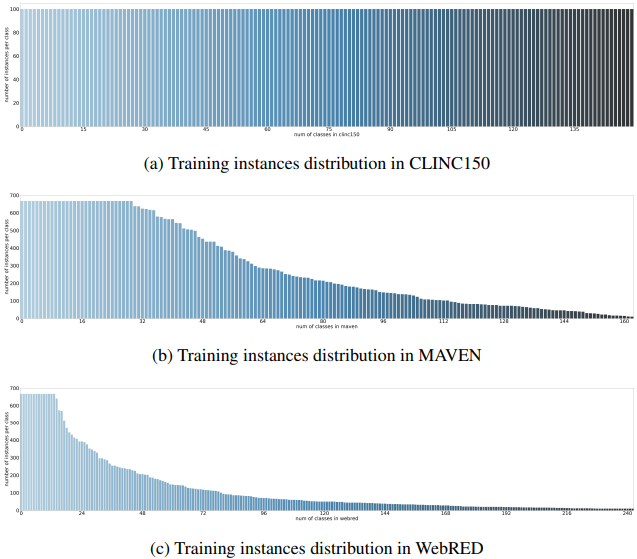The vertical axis shows the number of data per class and the horizontal axis shows the number of classes.

### experimental results

Experiments were conducted in each setting and the accuracy obtained is summarized in the table below.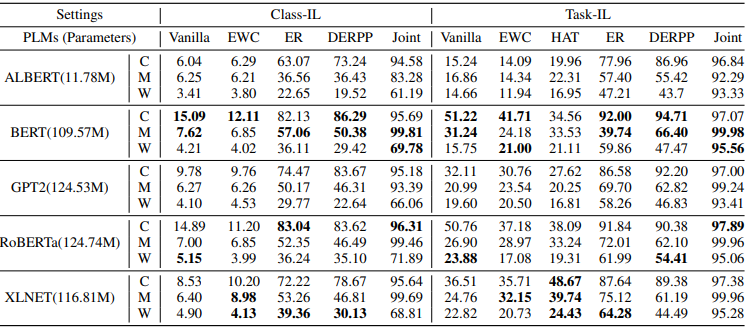As can be seen by comparing the Vanilla and Joint accuracy of each PLM, severe catastrophic forgetting occurs in the PLM. This is common for all datasets (C, M, W) and is especially more severe in the Class-IL setting. The results of the different continuation learning methods for each model and the comparison of the results of the different models for each continuation learning method are as follows.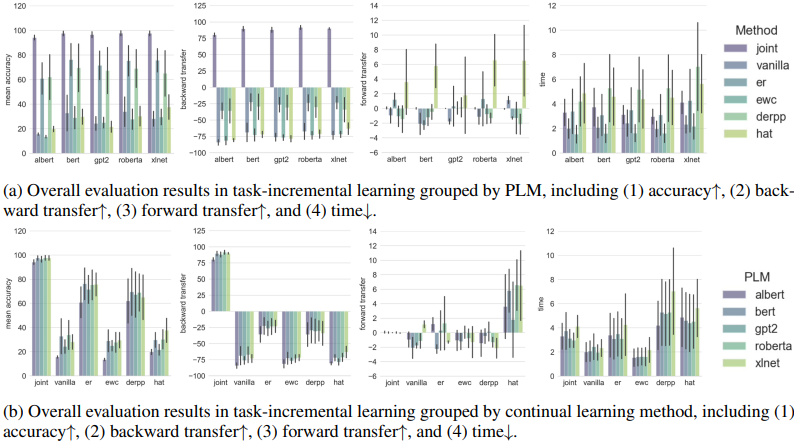In general, we found that BERT was the most robust among the PLMs and the rehearsal-based method was the most effective among the continuous learning methods.

To analyze the above results in more detail, the paper further investigates the following questions

• (1) What is happening in the black box of BERT during continuous learning?
• (2) What are the performance differences between PLMs and between layers within each PLM?
• (3) Why are rehearsal-based methods more robust than regularization-based methods?
• (4) At which tier does replay contribute the most?

To answer these questions, the experiment first measured the expressive power in each layer of BERT and each task in each layer of BERT (see original paper 4.1 for details).

The results are as follows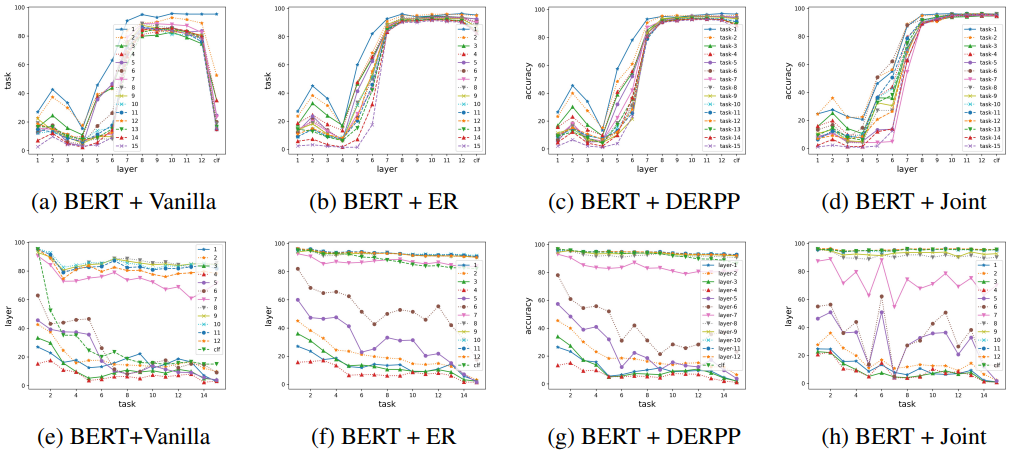The results show that catastrophic forgetting occurs in both the middle and final layers and that ER can suppress forgetting in the middle and final layers. For each PLM model, the representativeness of each layer in the Vanilla setting and when the ER method is applied with different buffer sizes are as follows.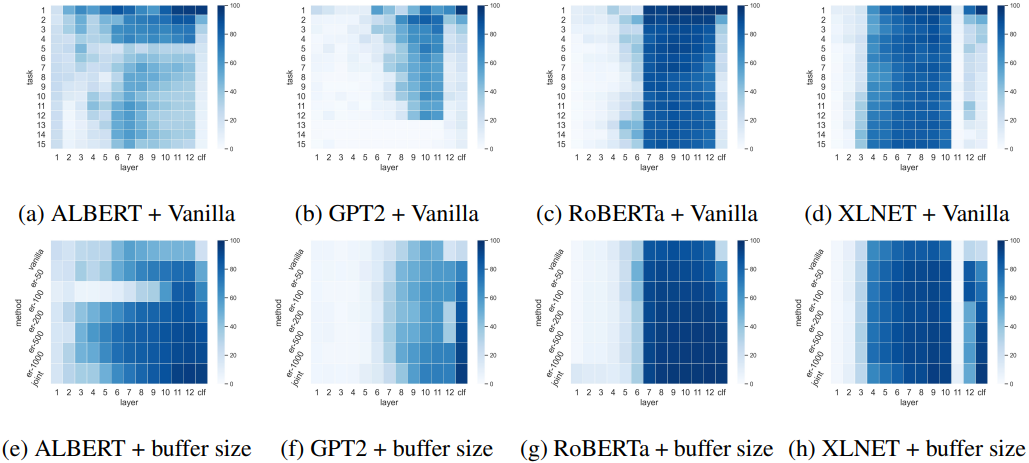Figures (a-d) show the representativeness of each task and layer in vanilla settings for each PLM, and (e-h) show the buffer size and representativeness of each layer when ER is applied (darker colors are better). Experimental results show that the robust and fragile layers differ depending on which PLM is used, and which layer's performance is also improved by replay.

For example, the hidden layer of ALBERT is more vulnerable than BERT and RoBERTa, possibly due to the parameter sharing mechanism. Regarding the impact of ER, XLNet improves the performance of the 12 and clf layers, while RoBERTa and GPT2 mainly improve the performance of the clf layer.

In general, the interesting result is that the robustness to continuous learning varies from layer to layer, and which layers are robust depends on the PLM architecture.

## summary

In this article, we presented a study that investigated the performance characteristics of a representative pre-learning language model combined with continuous learning and analyzed each layer within each language model.

The insights gained from this study are an important contribution to continuous learning with PLM.

If you have any suggestions for improvement of the content of the article,